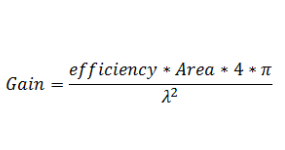#### Gain from aperture calculatorThis calculator calculates the expected gain for an aperture of a given area and efficiency. It can also invert the equation to solve for any of the other parameters. For instance, it can be used to calculate the aperture efficiency of a horn antenna.

The Calculator is based on the above formula.